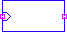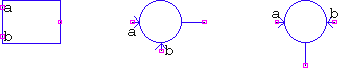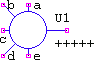These blocks make use of LTspice's builtin mathematical functions, plus a few others. The appearance of the symbols was made with the thought of uniformity and usability, which makes possible changing the function of the symbol on the fly, while still preserving the general distinct appearance. Some functions may seem unneeded duplicates, such as b-a, or b/a, but the purpose is the ability to keep the functionality without forcing the user to re-wire (this is mostly related to testing, where, too often, it becomes cumbersome).

For example, placing the Math1 symbol with its default abs value means the symbol will perform the abs() function; placing the Math2r symbol with x means multiplication. Both of these are easily recognizable in the schematic, and easily changed by either renaming or by right-clicking the symbol and selecting the desired entry from the drop-down menu. For example switching from sin() to cos() means renaming sin to cos (49.2kB webm); if b/a is needed instead of a/b, it can be achieved by simply renaming / to \ (63.7kB webm).

The models are divided in three: mathfunc1.sub, holding the one-input/variable functions (e.g. sin(x), x being the input), mathfunc2.sub, holding the two-inputs/variables functions (e.g. atan2(x,y), with x and y being the inputs), and mathfunc.sub, holding the oddball 5-input summer.

Sometimes, in addition to the usual input(s) as variable(s), there are some other parameters that can be defined, e.g. the atan2 block has the additional parameters a, b, and dc. All the blocks have floating input and output resistance, unless otherwise noted.

And, finally, in many cases the names of the functions are blatantly obvious and should not need explanations, yet the tables below display them. This has nothing to do with treating the reader as stupid, and all to do with consistency. There are no stand-alone examples here, but some usage can be seen in the examples section.

mathfunc1.sub
mathfunc2.sub
mathfunc.sub

### Subcircuits in mathfunc1.sub:

• All subcircuits from mathfunc1.sub make use of the Math1 symbol:. The input is marked with a squared arrow.
• Unlike mathfunc2.sub, the names of the functions are the same as the name given in the subcircuit definitions.
• Some common parameters, y, phi, have different meanings, shown in the Output column.
• The hidden parameters, where used, have common meanings:
 fmax Hz Estimated bandwidth for the implicit methods, default 1meg lim V Internal limits to avoid clipping, default 1g Cout F Output capacitance for aid in convergence, default 0 tripdv, tripdt V, s LTspice's specific for B-sources, defaults <0,0>
Function Subcircuit name Output Parameters used
1/x " " 1/V(in) lim, fmax
abs() " " abs(V(in)) lim, Cout
acos() " " acos(V(in) + phi) phi, tripdv, tripdt
acosh() " " acosh(V(in)) tripdv, tripdt
asin() " " asin(V(in) + phi) phi, tripdv, tripdt
asinh() " " asinh(V(in)) tripdv, tripdt
atan() " " atan(V(in) + phi) phi, tripdv, tripdt
atanh() " " atanh(V(in)) tripdv, tripdt
ceil() " " ceil(V(in)) tripdv, tripdt
cos() " " cos(V(in) + phi) phi, tripdv, tripdt
cosh() " " cosh(V(in)) tripdv, tripdt
exp() " " exp(V(in)) tripdv, tripdt
floor() " " floor(V(in)) tripdv, tripdt
int() " " int(V(in)) tripdv, tripdt
ln() " " loge(V(in)) tripdv, tripdt
log() " " loge(V(in)) tripdv, tripdt
log10() " " log10(V(in)) tripdv, tripdt
mod1() " " mod(V(in), y) y, tripdv, tripdt
pow() " " pow(V(in), y) y, tripdv, tripdt
pwr() " " pwr(V(in), y) y, tripdv, tripdt
pwrs() " " pwrs(V(in), y) y, tripdv, tripdt
quant() " " int(V(in)*y)/y y, tripdv, tripdt
round() " " round(V(in)) tripdv, tripdt
sgn() " " sgn(V(in) + y) y, tripdv, tripdt
sin() " " sin(V(in) + phi) phi, tripdv, tripdt
sinh() " " sinh(V(in)) tripdv, tripdt
sqrt() " " V(in) lim, fmax
tan() " " tan(V(in) + phi) phi, tripdv, tripdt
tanh() " " tanh(V(in)) tripdv, tripdt
u() " " if(V(in)+y>0, 1, 0) y, Cout, tripdv, tripdt
uramp() " " if(V(in)+y>0, V(in)+y, 0) y, Cout, lim

TOP

### Subcircuits in mathfunc2.sub:

• mathfunc2.sub makes use of three symbols:• The subcircuit names for the arithmetic and logic functions are different than the functions themselves, but chosen such as to be (hopefully) easily recognizable.
• The same notes about parameters are valid here, too.

Function Subcircuit name Output Parameters used
a+b + V(a) + V(b)
a-b - V(a) - V(b)
b-a | V(b) - V(a)
a*b * V(a)*V(b) lim
a/b / V(a)/V(b) lim, fmax
b/a \ V(b)/V(a) lim, fmax
a==b ~~[note] if(V(a)==V(b), 1, 0) tripdt
a!=b !~[note] if(V(a)!=V(b), 1, 0) tripdt
atan2(a,b) atan2 atan2(sgn(a)*V(a), sgn(b)*V(b)) + dc a, b, dc, tripdv, tripdt
hypot(a,b) hypot hypot(V(a), V(b)) lim, fmax
max(a,b) max max(V(a), V(b)) lim
min(a,b) min min(V(a), V(b)) lim
mod(a,b) mod mod(V(a), V(b)) tripdv, tripdt
[note] Names with "=" won't be recognized by LTspice, and using "==", or "!=", will make the names visible in the list, but not usable. Thus, "~~" and "!~" were chosen, hopefully, as visually and functionally similar alternatives.
TOP

### Subcircuits in mathfunc.sub:

mathfunc.sub holds what is meant to be all possible sum/difference combinations (except nulls) for a 5-input symbol:Unused inputs must be grounded!

```+++++
-++++
+-+++
++-++
+++-+
++++-
--+++
-+-++
-++-+
-+++-
+--++
+-+-+
+-++-
++--+
++-+-
+++--
---++
--+-+
--++-
-+--+
-+-+-
-++--
+---+
+--+-
+-+--
++---
----+
---+-
--+--
-+---
+----
-----```
TOP

The README file lists all the files inside the respective archive:
Math.zip (10308 B)
MD5=d29cec2451db47f0c246516643bc0da1
SHA256=2d1290322f1be48117d63df8d500a7b2db14044b423641b5bddd3dacef65d740
TOP